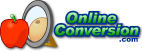## Welcome to OnlineConversion.com

At what temperature are Celsius and Fahrenheit the same?

The formulas for converting between degree Celsius and degree Fahrenheit are:

°F = (°C * 9/5) + 32
°C = (°F - 32) * 5/9

To find the temperature when both are equal, we use an old algebra trick and just set ºF = ºC and solve one of the equations.

°C = (°C * 9/5) + 32
°C - (°C * 9/5) = 32
-4/5 * °C = 32
°C = -32 * 5/4
°C = -40

°F = (°F * 9/5) + 32
°F - (°F * 9/5) = 32
-4/5 * °F = 32
°F = -32 * 5/4
°F = -40

So the temperature when both the Celsius and Fahrenheit scales are the same is -40 degrees.### BookMark Us

It may come in handy.

Check out our Conversion Software for Windows.Can't find something?
Try searching.

Are you bored?
Try the Fun Stuff.